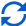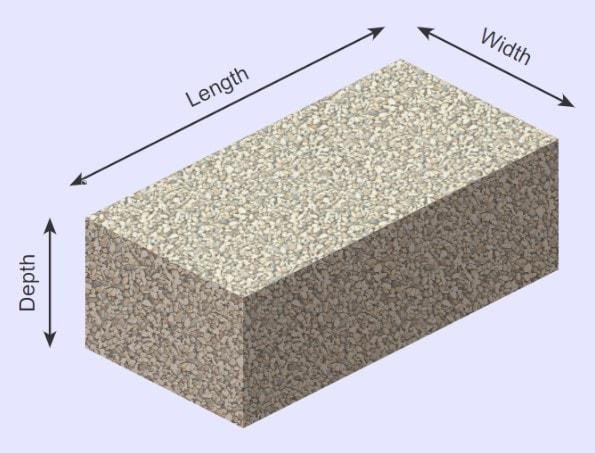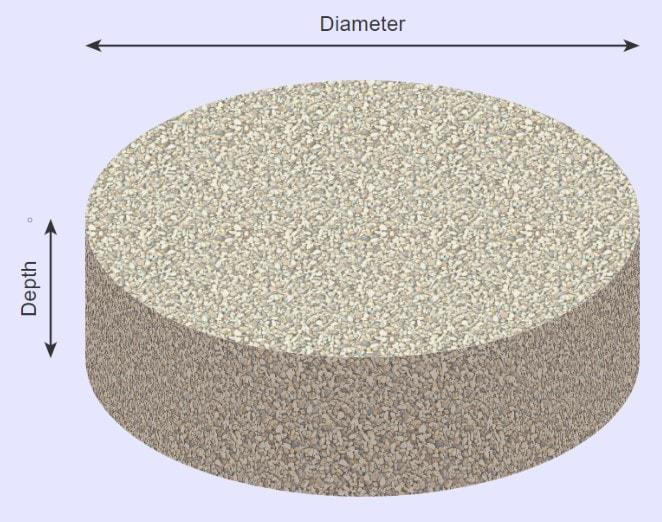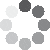Enter parameters Rectangular area
Enter parameters
LENGTH, WIDTH & DEPTH
Length:
ft
• inches (in)
• feet (ft)
• yards (yd)
• centimeters (cm)
• meters (m)
Width:
ft
• inches (in)
• feet (ft)
• yards (yd)
• centimeters (cm)
• meters (m)
Depth:
in
• inches (in)
• feet (ft)
• yards (yd)
• centimeters (cm)
• meters (m)
Density:
105 - Gravel lb/ft³
• Gravel - 105 lb/ft³
• Concrete - 150 lb/ft³
• Limestone - 160 lb/ft³
• Sandstone - 145 lb/ft³
• Granite - 168 lb/ft³
• Marble - 160 lb/ft³
• Slate - 168 lb/ft³
• Basalt - 188 lb/ft³
• Crushed stone - 100 lb/ft³
• Custom
Price per unit of mass:
t
• pounds (lb)
• US short tons (t)
• Imperial-Long tons (long t)
• kilograms (kg)
Price per unit of volume:
yd³
• cubic yards (yd³)
• cubic feet (ft³)
• cubic inches (in³)
• cubic centimeters (cm³)
• cubic meters ()Result
Area:
0
ft²
• square feet (ft²)
• square inches (in²)
• square yards (yd²)
• square miles (mi²)
• acres (ac)
• square centimeters (cm²)
• square meters ()
Volume:
0
yd³
• cubic yards (yd³)
• cubic feet (ft³)
• cubic inches (in³)
• cubic centimeters (cm³)
• cubic meters ()
Weight:
0
t
• pounds (lb)
• US short tons (t)
• Imperial-Long tons (long t)
• kilograms (kg)
Cost:
$Enter parameters Diameter: ft • inches (in) • feet (ft) • yards (yd) • centimeters (cm) • meters (m) Depth: in • inches (in) • feet (ft) • yards (yd) • centimeters (cm) • meters (m) Density: 105 - Gravel lb/ft³ • Gravel - 105 lb/ft³ • Concrete - 150 lb/ft³ • Limestone - 160 lb/ft³ • Sandstone - 145 lb/ft³ • Granite - 168 lb/ft³ • Marble - 160 lb/ft³ • Slate - 168 lb/ft³ • Basalt - 188 lb/ft³ • Crushed stone - 100 lb/ft³ • Custom Price per unit of mass: t • pounds (lb) • US short tons (t) • Imperial-Long tons (long t) • kilograms (kg) Price per unit of volume: yd³ • cubic yards (yd³) • cubic feet (ft³) • cubic inches (in³) • cubic centimeters (cm³) • cubic meters ()Result Area: 0 ft² • square feet (ft²) • square inches (in²) • square yards (yd²) • square miles (mi²) • acres (ac) • square centimeters (cm²) • square meters () Volume: 0 yd³ • cubic yards (yd³) • cubic feet (ft³) • cubic inches (in³) • cubic centimeters (cm³) • cubic meters () Weight: 0 t • pounds (lb) • US short tons (t) • Imperial-Long tons (long t) • kilograms (kg) Cost:$(6 votes, average: 5.00 out of 5)Loading...
Similar Calculators:
Real-time graphics. Make the calculations and see the changes.
Real-time graphics. Make the calculations and see the changes.
Embed
Similar Calculators:Contents:

## What is the stone calculator?

Estimating the amount of stone your outdoor landscaping project will require can be difficult.

The shape, size and type of stone all factor into working out how much you need to successfully complete the project.

If you’re not sure how to calculate volume or cost then scroll down for more information.

Otherwise, simply type your dimensions of the stone into the calculator.

Our bulk material calculator is a powerful tool that will help you calculate the volume of stone required.

In addition, if you know the density of the stone and cost per unit mass/volume, then you can also calculate the total cost.

Coupled with a little preparation, this calculator will ensure that your project will be a walk in the park!

## How to calculate how much stone do you need?

Calculate the volume of stone by entering your dimensions in imperial units (inches, feet or yards) or metric units (centimeters or meters).

The calculator will take care of any unit conversions so you don’t need to!

### Rectangular AreaThe total volume of a rectangular prism is calculated by:

$$Volume = Length \times Width \times Depth$$

### Circular AreaThe total volume of a circular prism is calculated by:

$$Volume = \pi\ \times \biggl({Diameter \over 2}\biggr)^2 \times Depth$$

You may be asking, what is density?

Density is a quantity describing how heavy an object is per unit of volume, for example number of pounds per cubic feet.

$$Density\,lb/ft^3 = {Mass\,(lb) \over Volume\,(ft^2)}$$

Our calculator has some density values for certain materials (gravel, concrete, limestone etc).

If you are using a different material and know its density you can enter this under the custom option.

If you have the price of the stone per unit mass (e.g. cost per pound) or price per volume (e.g. cost per cubic feet) you can work out the total cost.

$$Cost = Weight \times Price\,per\,unit\,mass$$

or

$$Cost = Volume \times Price\,per\,unit\,volume$$

Confused? 😕

See below for two examples. Otherwise, enter your measurements and values in our online calculator!

## Calculation examples

• Rectangular Area with Crushed Gravel (105 lb/ft³) and Price Per Unit Mass

Let’s say I need crushed gravel for part of my driveway which measures 4ft long, 2ft wide and 9in (0.75ft) deep.

Let’s also say that the selected gravel costs $50 per ton. I would enter these measurements for length, width, depth and density which would then perform the following calculation to work out the cost for covering the section of driveway: $$Volume = Length \times Width \times Depth = 4 \times 2 \times 9 = 0.222\,yd^3$$ $$Weight = Density \times Volume = 105\,lb/ft^3 \times 0.222\,yd^3 = 0.315\,t$$ $$Cost = Weight \times Price\,per\,unit\,mass = 0.315\,t \times 50\,/t = 15.75$$ • Circular Area with Custom Stone and Price Per Unit Mass Let’s say I have a stone not listed in the options for density, with a diameter of 10 feet at a depth of 12 inches. The density of the stone is 100 lb/ft³, and it costs$10 per yd³.

I enter these values in the calculator which would perform the following calculations:

$$Volume = \pi\ \times \biggl({Diameter \over 2}\biggr)^2 \times Depth = \pi\ \times \biggl({10 ft \over 2}\biggr)^2 \times 12\,in = 2.909 ft^3$$

$$Cost = Volume \times Price\,per\,unit\,volume = 2.909 ft^3 \times 10\,/t = 29.09$$

Note that in the above two examples, the units of each measurement are not the same (e.g. density is in pounds per cubic feet and volume is given in cubic yards).

There are multiple options for the units of each measurement that are available to use.

For example, you can enter measurements of length in inches (in), feet (ft), yards (yd), centimeters (cm) or meters (m).

Simply select the correct unit in the drop-down area and our calculator will do the rest. 😃

Embed this calculator on your site!Add live graphics
Copied to clipboard! PreviewWhat's wrong?
Minimum 5 characters!